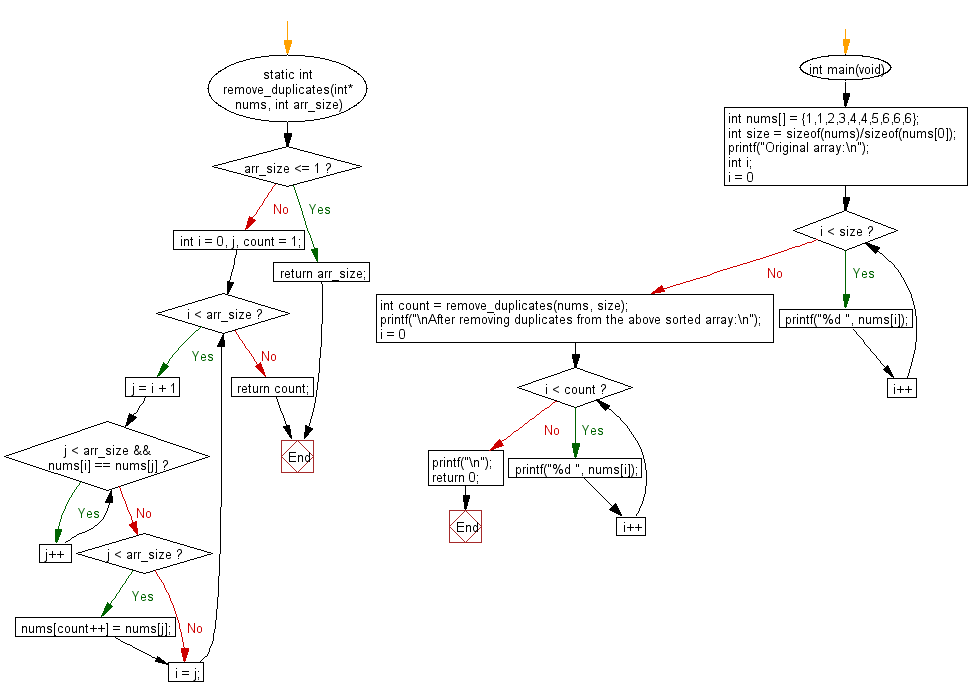﻿ C Program: Remove duplicates from an array of integers# C Exercises: Remove the duplicates from a given array of integers

## C Programming Practice: Exercise-12 with Solution

Write a C programming to remove the duplicates from a given array of integers.

C Code:

``````#include <stdio.h>
#include <stdlib.h>
static int remove_duplicates(int* nums, int arr_size)
{
if (arr_size <= 1) {
return arr_size;
}

int i = 0, j, count = 1;
while (i < arr_size) {
for (j = i + 1; j < arr_size && nums[i] == nums[j]; j++) {}
if (j < arr_size) {
nums[count++] = nums[j];
}
i = j;
}

return count;
}

int main(void)
{
int nums[] = {1,1,2,3,4,4,5,6,6,6};
int size = sizeof(nums)/sizeof(nums);
printf("Original array:\n");
int i;
for (i = 0; i < size; i++) {
printf("%d ", nums[i]);
}
int count = remove_duplicates(nums, size);
printf("\nAfter removing duplicates from the above sorted array:\n");
for (i = 0; i < count; i++) {
printf("%d ", nums[i]);
}
printf("\n");
return 0;
}
``````

Sample Output:

```Original array:
1 1 2 3 4 4 5 6 6 6
After removing duplicates from the above sorted array:
1 2 3 4 5 6
```

Pictorial Presentation:Flowchart:## C Programming Code Editor:

What is the difficulty level of this exercise?

Test your Programming skills with w3resource's quiz.

﻿

## C Programming: Tips of the Day

Maximum value of int:

In C:

```#include <limits.h>
then use
int imin = INT_MIN; // minimum value
int imax = INT_MAX;```

or

```#include <float.h>

float fmin = FLT_MIN;  // minimum positive value
double dmin = DBL_MIN; // minimum positive value

float fmax = FLT_MAX;
double dmax = DBL_MAX;```

Ref : https://bit.ly/3fi8yk9